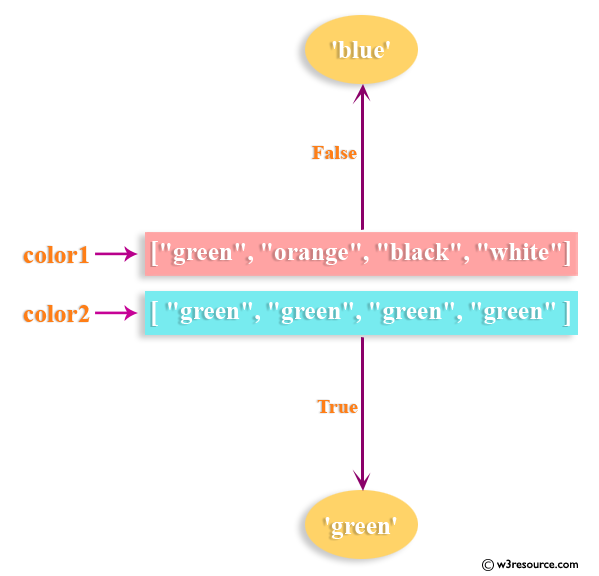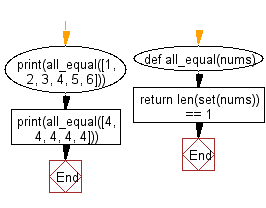﻿ Python: Check whether all items of a list is equal to a given string - w3resource# Python: Check whether all items of a list is equal to a given string

## Python List: Exercise - 57 with Solution

Write a Python program to check if all items of a given list of strings is equal to a given string.Sample Solution-1:

Python Code:

``````color1 = ["green", "orange", "black", "white"]
color2 = ["green", "green", "green", "green"]

print(all(c == 'blue' for c in color1))
print(all(c == 'green' for c in color2))
```
```

Sample Output:

```False
True
```

## Visualize Python code execution:

The following tool visualize what the computer is doing step-by-step as it executes the said program:

Sample Solution-2:

Checks if all elements in a list are equal:

Use set() to eliminate duplicate elements and then use len() to check if length is 1.

Python Code:

``````def all_equal(nums):
return len(set(nums)) == 1
print(all_equal([1, 2, 3, 4, 5, 6]))
print(all_equal([4, 4, 4, 4, 4]))
```
```

Sample Output:

```False
True
```

Flowchart:## Visualize Python code execution:

The following tool visualize what the computer is doing step-by-step as it executes the said program:

Python Code Editor:

Have another way to solve this solution? Contribute your code (and comments) through Disqus.

What is the difficulty level of this exercise?

Test your Python skills with w3resource's quiz

﻿

## Python: Tips of the Day

Floor Division:

When we speak of division we normally mean (/) float division operator, this will give a precise result in float format with decimals.

For a rounded integer result there is (//) floor division operator in Python. Floor division will only give integer results that are round numbers.

```print(1000 // 300)
print(1000 / 300)```

Output:

```3
3.3333333333333335```